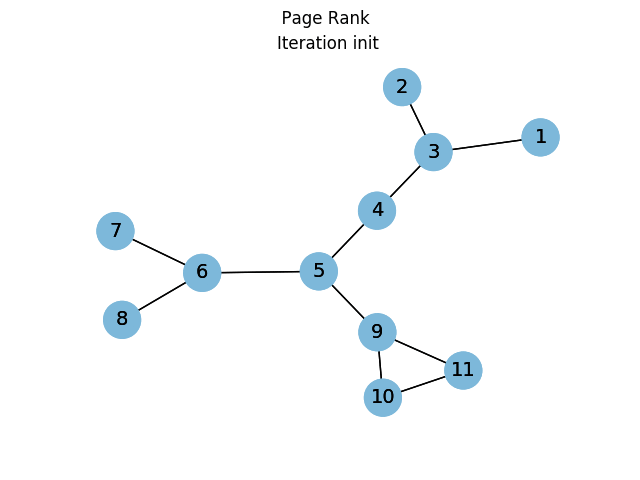# Visualizing PageRank using networkx, numpy and matplotlib in python

## March 07, 2020

Today I wanted to understand how the PageRank algorithm works by visualizing the different iterations on a gif. But to make the exercise more complicated (interesting ;-)), I also wanted to implement my own PR algorithm using matrix formulation.

## Implementation

In terms of implementation, I decided to rely on the networkx representation of graphs and their methods such as adjacency_matrix. The graph is created using for instance:

import networkx as nx



Then, we just need to iterate for a maxium of max_iter, or until the desired mean error is reached. At each step, the PageRank is updated with:

pr = d * weight.dot(pr) + (1-d)/N


Where the weight matrix is a NxN matrix whose ij element is the weight between node i and j (1/deg(j)).

The full code is reproduced here:

import numpy as np
def page_rank(G, d=0.85, tol=1e-2, max_iter=100):
"""Return the PageRank of the nodes in the graph.

:param dict G: the graph
:param float d: the damping factor
:param flat tol: tolerance to determine algorithm convergence
:param int max_iter: max number of iterations
"""
nodes = G.nodes()
out_degree = matrix.sum(axis=0)
weight = matrix / out_degree
N = G.number_of_nodes()
pr = np.ones(N).reshape(N, 1) * 1./N

# need to repeat the initial step twice
# for matplotlib animation
yield nodes, pr, "init"
yield nodes, pr, "init"

for it in range(max_iter):
old_pr = pr[:]
pr = d * weight.dot(pr) + (1-d)/N
yield nodes, pr, it
err = np.absolute(pr - old_pr).sum()
if err < tol:
return pr
#raise Exception(f'PageRank failed after max iteration = {max_iter} (err={err} > tol = {tol})')


The resulting values for PageRank using this implementation are compatible with the ones obtained with the networkx implementation:

# Custom page rank:
[[0.05427205 0.14652879 0.05427205 0.08972923 0.12543861 0.1398845
0.11906867 0.0530322  0.0530322  0.08237085 0.08237085]]
# network page rank:
{'1': 0.05472174724228272, '3': 0.14500947724655655, '2': 0.05472174724228272, '4': 0.09022185969480352, '5': 0.1252959204066461, '6': 0.13952052031260131, '9': 0.1192351424187364, '7': 0.05316762893415654, '8': 0.05316762893415654, '10': 0.0824691637838888, '11': 0.0824691637838888}



This function can then be used within a matplotlib animation to visualize the rank evolution until convergence.

## Visualization

Since the function was implemented as a generator, yielding the current ranks at each iteration, we can directly use it in a FunctionAnimation. We just need an update function, that will actually draw the graph with node colors depending on the rank (the darker the blue, the highest the rank):

import matplotlib.pyplot as plt
from matplotlib.animation import FuncAnimation

def update(r):
res_nodes, res_values, it = r
res_values = np.asarray(res_values).ravel()
plt_nodes = nx.draw_networkx_nodes(
G, pos,
ax=ax,
nodelist=res_nodes,
node_color=res_values,
alpha=1,
node_size=700,
cmap=plt.cm.Blues,
vmin=0,
vmax=0.2
)
ax.axis("off")
ax.set_title(f"Iteration {it}")
nx.draw_networkx_edges(G, pos)
nx.draw_networkx_labels(G, pos, font_size=14)
return [plt_nodes, ]

f, ax = plt.subplots()
ani = FuncAnimation(
f,
update,
frames=page_rank(G),
interval=1000,
blit=True
)
f.suptitle(f"  Page Rank")
ani.save("graph_pr.gif", writer='imagemagick')


The result of this code is the following animated gif, where one can clearly see the rank distribution, especially during the first steps: